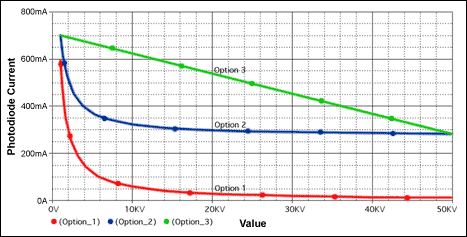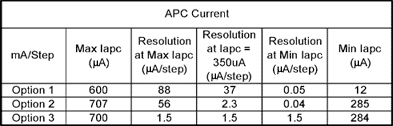# Interfacing the DS1859 Digitally Controlled Resistors to the MAX3735 Laser Driver

### Abstract

This article describes three options for interfacing the DS1859 Digitally Controlled Resistors to the MAX3735 Laser Driver. Included is a new technique using an op amp that gives a linear transfer function. It can be adjusted for any arbitrary current range. It can even generate currents larger than those flowing in the DS1859 potentiometer at minimum resistance. These techniques can be applied to other parts as well.

### Introduction

The designer has several options for interfacing the DS1859 digitally controlled resistors to the MAX3735 laser driver. The simplest option is to connect the variable resistors of the DS1859 directly to the MODSET and APCSET pins of the MAX3735. This will be called Option 1 (Figure 5). The advantage of this approach is that it requires no extra parts. The disadvantage is nonlinearity with low resolution at high currents.

Another option for this interface is to use a resistor divider to rescale the current control range. We will call this Option 2 (Figure 6). A smaller range will give better resolution than Option 1, but still suffers from nonlinearity.

A third option is to use an op amp to linearize the current vs. potentiometer value transfer function. This is Option 3 (Figure 7). In addition to giving better resolution, this third technique can be scaled for any arbitrary current range. It can even generate currents larger than those flowing in the DS1859 pot at minimum resistance. The only limitation to Option 3 is the current drive capability of the op amp. Option 3 is a new technique and will be described in detail here, along with all required design information.

The examples in this article are based on the reference design: "HFRD-04.0: 2.7Gbps, 1310nm Small Form Factor Pluggable (SFP) Transceiver." These techniques can also be applied to many other parts besides the DS1859 and MAX3735.

All three of these techniques were simulated on PSPICE (Figures 1/2/3/4). Schematics, simulation results, and summaries are included in this article.

### PSPICE Simulation ResultsFigure 1. PSPICE results: Modulation current vs. potentiometer value.Figure 2. Summary: Modulation current vs. potentiometer value.Figure 3. PSPICE results: Photodiode current vs. potentiometer value.Figure 4. summary: Photodiode current vs. potentiometer value.Figure 5. Option 1 schematic.

The MAX3735 (Figure 5) uses a current mirror to generate the modulation and APC set value. A 1.2V bandgap reference sets the voltage at the MODSET and APCSET nodes. The resistance to ground at these nodes defines the mirror current. The MODSET current is multiplied by the mirror gain of 270 to give the final modulation current value. The APC control loop will servo the laser power, until the monitor photodiode current equals that defined by APCSET. The monitor photodiode current is ½ the value at APCSET. (The MAX3735 data sheet showing APCSET gain of 1 is in error.)

Option 1
The simplest interfacing scheme connects the DS1859 directly to MODSET and APCSET. This gives a current proportional to the inverse of resistance. This nonlinear inverse function means that you have very low resolution at high currents and very high resolution at low currents. Another problem is that the modulation current is above the recommended maximum of 60mA for the first 10% of the DS1859's range. Because these high current values could accidentally be selected during programming or testing, you need to ensure that they will not damage any components or cause an eye-safety hazard.

If you can work around all these limitations, this would be your lowest cost option.Figure 6. Option 2 schematic, divider.

Option 2
In this option a resistor divider is added to the MODSET and APCSET pins (Figure 6). The total current flowing through this divider establishes a minimum current value. The value of the upper resistor (R1 and R5) establishes a maximum current value. Proper selection of these values can limit the current range and give greater resolution in the range of interest. The effects of these resistors are interdependent on each other and the value of the variable resistor. For more information on design techniques for this option see HFAN-02.3.3, "Optimizing the Resolution of Laser Driver Current Settings Using a Linear Digital Potentiometer."Figure 7. Option 3 schematic, Op amp circuit.

Option 3
In Options 1 and 2 the current through the potentiometer is proportional to 1/R. This inverse function is the cause of the nonlinearity problems. If the potentiometer is put in the feedback loop of an op amp, then gain is a linear function of potentiometer value. If this op amp amplifies a reference voltage by this linear gain, then the op amp's output voltage is also a linear function of potentiometer value. This voltage can then be used to generate a current that is a linear function of potentiometer value. Once you have a current that is a linear function of R, all the 1/R nonlinearity problems go away.

The operation of the circuit (Figure 7) can be understood as follows. (Reference designations are for the MODSET example.) R1 and R2 generate a reference voltage at the noninverting input of op amp U3 (0.2V in the example). The current through this divider also contributes to MODSET currents, so it must be accounted for in our design equations. The reference voltage is multiplied by the op amp gain, 1+Rds1859/R3. The current through R4 is determined by the voltage at MODSET (1.2V), minus the voltage at the op amp output, divided by the value of R4.

Option 3 Design
The following sections contain all the equations necessary for designing the MODSET and APCSET op amp circuits. Before you can use these equations, you must make the following engineering decisions.

Op amp selection
The op amp must have good DC performance and be small and inexpensive. The MAX4245 single op amp in a SC70 package and the MAX4246 dual op amp in a SOT23 package meet these requirements. They have 1.5mV (max) input offset voltage, 6nA (max) input offset current, and 50nA (max) bias current.

Voltage at op amp noninverting input
This voltage must be large enough to minimize the effects of op-amp offset voltage. PSPICE worst-case analysis of the above circuits indicated values above 100mV would be satisfactory. 200mV was chosen for this example.

Max and min current ranges
You need to determine what current ranges you want for MODSET and APCSET. These should be large enough to cover the effects of initial tolerance, temperature, and aging of all components. The values in this example were chosen to be similar to those in the HFRD-04.0 reference design.

Voltage divider top resistor value
The voltage-divider top resistors, R1 and R5, need to be high enough so that the divider current is less than the minimum desired current value at MODSET or APCSET. The 49.4K values chosen in this example give 20µA currents, well below the required minimum current values.

Once you make the above decisions, insert your results into the following equations to get the remaining resistor values. The values given by the equations probably will not correspond to standard values, so choose something close. Go back and recalculate, simulate, or build the circuit using the actual resistor values to ensure that it gives you the desired current ranges. As with all electronic circuits, these designs should be thoroughly tested before being put into production.